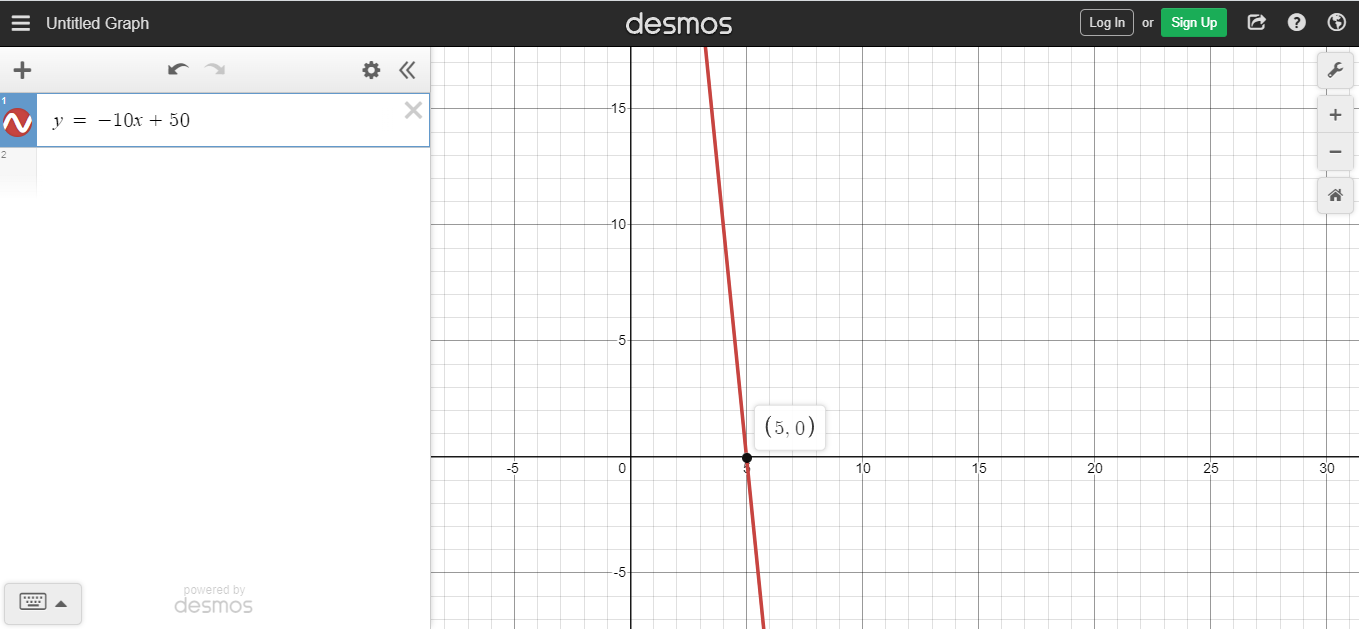# Linear Equations From Table of Values

in STEMGeeks7 months ago

Hi everyone. In this post, I cover the math topic of determining linear equations from a table of values. As long a table of values meets some conditions we can determine a linear function that passes these points.Pixabay Image Source

## Topics

• What Is A Linear Function?
• Determining Linear Functions From A Table Of Values

## What Is A Linear Function?

A linear function is a function where the inputs correspond in such a way where the rate of increase or decrease stays the same every time the input value increases by one. The general form of the linear function is: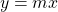where

• x is the independent variable or input
• m is the slope or the rate of increase/decrease for every 1 unit increase in x
• y is the output from x given a slope m

Example One

One apple costs 50 cents. Two apples would cost 100 cents. For every 1 apple increase the cost for the apples goes up by 50 cents. Let `n` be the number of apples. The cost for the apples (A) would be `A = 0.5 x n`.

Example Two

Consider a second example. A taxi fare has a cost for going into the cab and a variable cost that depends on the number of kilometres traveled. I am using price data from numbeo.com for Toronto. Getting in a taxi costs \$4.25 CAD and it costs \$2.00 CAD for every kilometre traveled.

Let `y` be the cost of taxi trip and let `x` be the number of kilometres travelled. The linear function for this Toronto taxi fare case would be: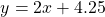The 4.25 CAD amount is called the y-intercept. It is the y-value when `x = 0`. In this case, \$4.25 CAD would be the entry cost or base fee of the taxi ride. Each kilometre travelled costs \$2 each on top of the \$4.25 initial cost.Pixabay Image Source

## Determining Linear Functions From A Table Of Values

What if all you have are a table of values? From the table of values, you would need to determine the slope and the y-intercept to obtain a corresponding linear function.

Here are a few examples. These two examples are not too difficult (I think).

Example One

Given a table of values, what is the equation of the line that passes these points?

xy
-2-11
-1-6
0-1
14
29

For every 1 unit increase for `x`, the value of `y` increases by 5. The rate of change or slope is 5. This five is the slope or the value for m.

With the equation of a line, we have: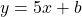The y-intercept can be quickly determined from the table of values. It is the value for y when `x = 0`. Negative one would be the y-intercept.

For this example, the equation of the line is `y = 5x -1`. A graph of this line would look something like this Desmos screenshot.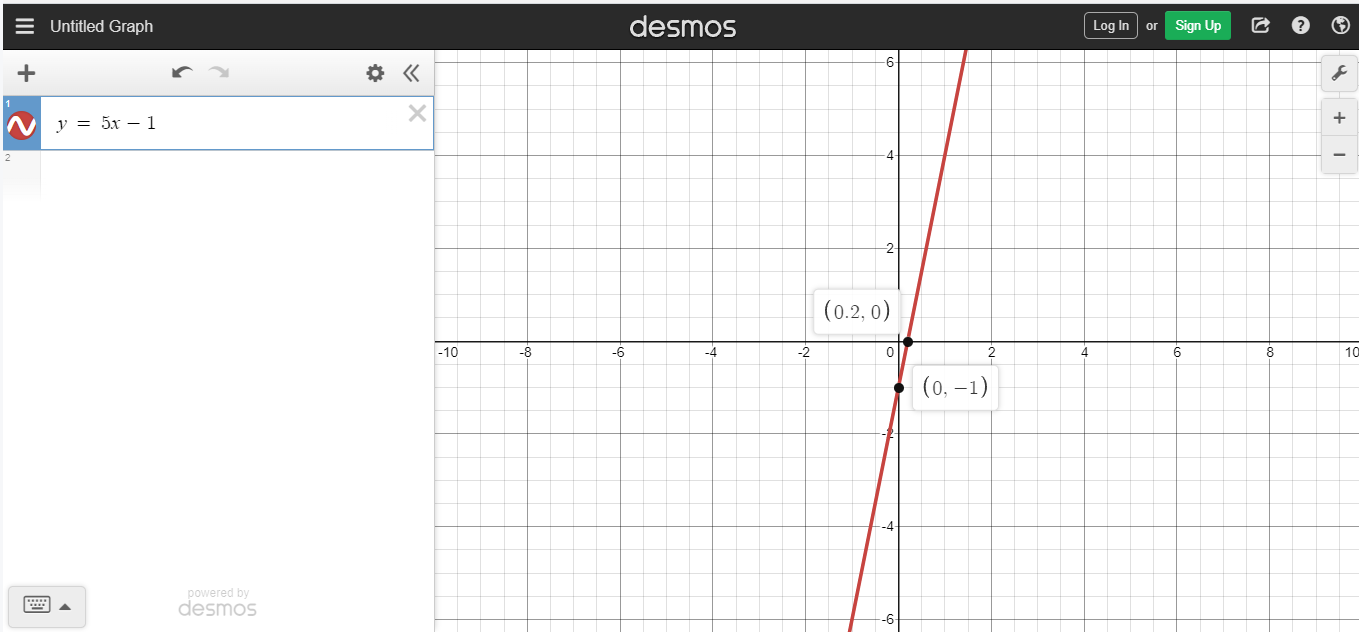Example Two

Determine the equation of the line that passes these 3 points given in the table below.

xy
140
230
320

From the table, a one unit increase for `x` corresponds to a decrease of 10 for `y`. The slope here for the equation of a line is just negative ten.

So far we have: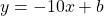The value of the y-intercept `b` needs to be determined. Select an (x, y) point from table of values, substitute accordingly for `x` and `y` and then solve for `b`. I choose `(1, 40)` as my `(x, y)` point.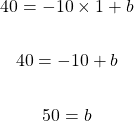With the value of `b` being 50 and a slope of `-10`, the equation of the line that passes through the 3 points from the table is `y = -10x + 50`.